# Triangle-packing vs triangle edge-transversal.

 Importance: Medium ✭✭
 Author(s): Tuza, Zsolt
 Subject: Graph Theory » Extremal Graph Theory
 Keywords:
 Posted by: fhavet on: March 6th, 2013
Conjecture   Ifhas at mostedge-disjoint triangles, then there is a set of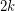edges whose deletion destroys every triangle.

This conjecture may be rephrased in terms of packing and edge-transversal. A triangle packing is a set of pairwise edge-disjoint triangles. A triangle edge-tranversal is a set of edges meeting all triangles. Denote the maximum size of a triangle packing inbyand the minimum size of a triangle edge-transversal ofby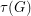. Clearly. The conjecture translates in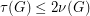.

This conjecture, if true, is best possible as can be seen by taking, say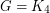or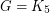. Trivially,, since the set of edges of a maximum triangle packing is a triangle edge-transversal. Haxell [H] proved that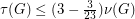edges whose deletion destroys every triangle.

As usual, one can define fractional packing and fractional transversal. Let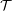be the set of triangles of. A fractional triangle packing is a function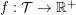such thatfor every edge. A fractional triangle edge-transversal is a function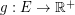such that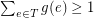for every triangle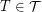. We denote by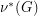the maximum of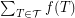over all fractional triangle packing and by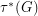the minimum of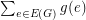over all fractional edge-transversals. By duality of linear programming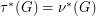. Krivelevich [K] proved two fractional versions of the conjecture: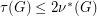and.

## Bibliography

[H] P.Haxell, Packing and covering triangles in graphs, Discrete Mathematics 195 (1999), no. 1–3, 251–254.

[K] M. Krivelevich, On a conjecture of Tuza about packing and covering of triangles Discrete Mathematics 142 (1995), 281-286.

*[T] Z. Tuza, A conjecture on triangles of graphs. Graphs Combin. 6 (1990), 373-380.

* indicates original appearance(s) of problem.